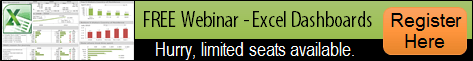## 15-significant-digit issue with SUMIF(S), COUNTIF(S), AVERAGEIF(S)Have you applied SUMIF or COUNTIF to a range that contains numbers with more than 15 digits stored as text? If you did or if you do, you’d better continue to read this as you’ve got wrong answers that you may not be aware of.  See example below:### Have you heard of “15 significant digits in Excel”?

Try to input any number with 16 digits ending with a non-zero number in Excel (Format the cell as number with no decimal). You will see that Excel converts the last digit to 0 without informing you.  😦  Simply put, all number at and beyond 16th digit will be converted into 0.  (Note: Excel does this with a purpose which is too comprehensive and beyond the scope of discussion (my knowledge) here. 😛  If you wish to know more, you may Google it.)

Nonetheless we may need to input a very large number and need all digits to be displayed in many cases.  Credit Card Number is a common example.  We can achieve this by putting an apostrophe coma ‘ in front of the number, e.g. ‘8888123456784321, so that Excel knows we are going to input Text instead of number.   See the screenshot below:A smart tag indicator telling you that the number input is stored as text.

As the number is being input as text, you do not expect the text to perform any calculation.  Logically, you do not add your Credit Card Number to get another Credit Card Number, do you? However, it is not uncommon to perform SUMIF(S), COUNTIF(S), AVERAGEIF(S) to them as they are supposed to be “text”.   And here comes the problem:As you see from the above, the functions SUMIF(S), COUNTIF(S), AVERAGEIF(S) give you incorrect answer which you do not expect.  It’s really a headache as you have checked that all the numbers were input as text. Putting =SUM(C4:C7) gives a result of 0, meaning that the contents in the range are text indeed.As discussed in SUM vs. + before, Excel converts number stored as text into number if you apply a mathematical operation to it directly.  So I tried:See?! The texts were converted back to numbers with only 15 significant digits, which is how Excel handles large number in the calculation.  Both “10000000000000511” were converted to “10000000000000500” and the result 20…1000 was returned.

Looking back to our problems, these commonly used Excel functions (SUMIF(S), COUNTIF(S), AVERAGEIF(S)) somehow convert number stored as text to number in the evaluation process.  In other words, these functions see “10000000000000511”, “10000000000000512”, “100000000000005xx” the same as they are eventually converted into 10000000000000500.We know the problem now. So what is the solution?

## Solution 1 – Array Formula

First, let’s see if Excel really treats “10000000000000511” and “10000000000000512” as the same?YEAH! The answer is NO! It means the function IF does not convert number stored as text to number; and it is able to treat them as different texts. Therefore C4:C7 = G1 returns an array of {FALSE;FALSE;TRUE;TRUE}.  So we can deploy the following as an alternative to COUNTIF

`=SUM(--(\$C\$4:\$C\$7=\$G\$1)) ‘CTRL SHIFT ENTER`

where

• C4:C7 = G1 evaluates each cell in the range with G1 (i.e “10000000000000512”) and returns an array of {FALSE;FALSE;TRUE;TRUE}
• The double – – turns TRUE to 1; FALSE to 0, returning an array of {0;0;1;1}
• Summing this resulting array gives you the same result as COUNTIF

By following the same logic, see a slightly different construction for SUMIF or AVERAGEIF can be built as follow:

`=SUM(IF(\$C\$4:\$C\$7=\$G\$1,\$D\$4:\$D\$7)) ‘CTRL SHIFT ENTER `

If you don’t like CTRL SHIFT ENTER, you may use SUMPRODUCT that can handle array

```=SUMPRODUCT(--(\$C\$4:\$C\$7=\$G\$1)) ‘as an alternative to COUNTIF
=SUMPRODUCT(--(\$C\$4:\$C\$7=\$G\$1),\$D\$4:\$D\$7) ‘as an alternative to SUMIF
```

## Solution 2 – Helper Column

I heard that. You want to stick to the original COUNTIF, SUMIF and you don’t mind having helping column. Simply concatenate the data with a Letter, e.g. = “R” & C4.  By doing so, the functions won’t have a chance to treat the range as number.Other than COUNTIF(S), SUMIF(S), AVERAGEIF(S), I do not know if there are other functions that would convert number stored as text to number for calculation.  Please share in comment if you know, or if you have encountered other issues with 15-significant-digit.An Excel nerd who just transition into a role related to data analytics at current company......😊 Recently in love with Power Query and Power BI.😍 Keep learning new Excel and Power BI stuffs and be amazed by all the new discoveries.
This entry was posted in Formula and tagged , , , , . Bookmark the permalink.

### 5 Responses to 15-significant-digit issue with SUMIF(S), COUNTIF(S), AVERAGEIF(S)

1.Hoang says:

Thank you very much. I have invoices countif and have errors. I did not know Excel have such issue.

Like

•MF says:

You are welcome. Glad It helps. Indeed Excel has many “interesting” behaviors . 😄

Like

2.ANA I Buscaglia says:

I have been using sumif formula for years without any issue until now….. I was you g nuts! Thanks for a great explanation and examples! This was a huge help!!

Like

•MF says:

You are welcome. Glad it helps .

Like

3.ANA I Buscaglia says:

Thank you SOOO Much!!! I Was going crazy trying to understand what was going on!!!!

Like

This site uses Akismet to reduce spam. Learn how your comment data is processed.